# Submanifold

In the narrow sense, an-dimensional topological submanifold of an-dimensional topological manifoldis a subset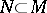which is an-dimensional manifold in the induced topology. The number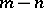is called the codimension of the submanifold. One most frequently encounters locally flat submanifolds, for which the identity imbedding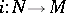is a locally flat imbedding. A subsetis a locally flat submanifold if for each point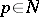there exists a neighbourhoodof this point intogether with local coordinates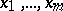in it such that in terms of these coordinatesis described by the equations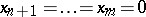.

In the broad sense, an-dimensional topological submanifold of an-dimensional topological manifoldis an-dimensional manifoldwhich, as a set of points, is a subset of(in other words,is a subset ofwith the structure of an-dimensional manifold) and for which the identity imbeddingis an immersion (cf. Immersion of a manifold). A submanifold in the narrow sense is a submanifold in the broad sense, while the latter is a submanifold in the narrow sense if and only ifis an imbedding in the topological sense (i.e. for each pointthere are arbitrarily small neighbourhoods inthat are intersections withof certain neighbourhoods in).

A piecewise-linear, analytic or differentiable submanifold (of class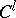,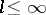) of a piecewise-linear, analytic or differentiable manifold(of class,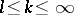) in the broad sense (or, correspondingly, in the narrow sense) is a subsethaving the structure of a piecewise-linear, analytic or differentiable manifold (of class), whereis a piecewise-linear, analytic or differentiable immersion (of class) (correspondingly, an imbedding). The definition of a differentiable submanifold of classis suitable also for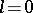, and it coincides in that case with the definition of a topological submanifold. It is usually understood that.

In the analytic and differentiable cases, the submanifold is always locally flat. Therefore, the definition of an analytic (differentiable) submanifold in the narrow sense is usually formulated from the start as an analytic (differentiable) form of the definition given in 1) for a locally flat submanifold by means of local coordinates, with the additional condition that the local coordinatesare analytic (differentiable of class). If a subsetsatisfies the latter definition, it is equipped in a natural way with the structure of an analytic (differentiable of class) manifold, andis an imbedding in the sense of the corresponding structure.

A piecewise-linear submanifold in the narrow sense can be locally represented as a subpolyhedron in the ambient manifold and is piecewise linearly equivalent to a simplex (cf. Simplex (abstract)). It is not always locally flat (although this is so for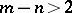); also, for such a manifold, the property of being locally flat in the topological sense does not coincide (at least directly) with the property of being locally flat in the piecewise-linear sense.

A simple modification of these definitions gives the definitions of: a submanifold with boundary; a submanifold of a manifold with boundary (in the topological situation, it is desirable to impose a restriction on the submanifold at the boundary of the ambient manifold, see ); a submanifold the various components of which may have different dimensions; a submanifold of a manifold of infinite dimension ; and a complex-analytic submanifold of a complex-analytic manifold.

The concept of a manifold in the narrow sense is a direct generalization of the concepts of a curve and a surface. A submanifold in the broad sense is used in the theory of Lie groups (where this concept was first introduced ), in differential geometry

and also in the theory of foliations (cf. Foliation).

In algebraic geometry, a submanifold (usually called a subvariety here) is a closed subset of an algebraic variety in the Zariski topology. This formalizes the idea that a subvariety is specified by algebraic equations. In addition to the transition fromto other fields, the change in the concept of a subvariety in this case is that one allows subvarieties with singularities.